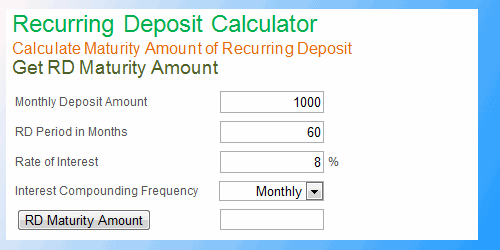Home        Online Calculators        Personal Finance        Corporate Finance        Spreadsheet Models        DerivativesAnnual Percentage Rate (APR): APR represents effective annualised interest rate of a borrowing factoring in all charges associated with the loan. In many jurisdictions, lenders are required by law to disclose APR of their loan products, as this indicator is supposed to make it easier for borrowers to compare lenders on true total cost basis. APR calculation is a bit complex; nevertheless it is not rocket science. We explain how to calculate APR in a spreadsheet. Read APR Calculation in Excel IRR Calculation: Internal Rate of Return (IRR) is an important investment appraisal tool to evaluate and compare return on investment with time value of money factored in. IRR is relatively simple to calculate in spreadsheet with Excel's built in function. However, when cash flows are at non-annual frequencies or non-uniform intervals, it gets tricky. Read about IRR Calculation in Excel.